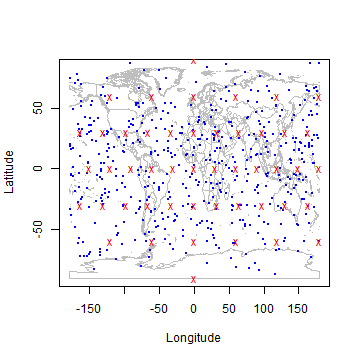Area of polygons and sampling¶

Area and perimeter of polygons¶

You can compute the area and perimeter of spherical polygons like this.

pol <- rbind(c(-120,-20), c(-80,5), c(0, -20), c(-40,-60), c(-120,-20))
areaPolygon(pol)
##  4.903757e+13
perimeter(pol)
##  27336557

Sampling longitude/latitude data¶

Random or regular sampling of longitude/latitude values on the globe needs to consider that the globe is spherical. That is, if you would take random points for latitude between -90 and 90 and for longitude between -180 and 180, the density of points would be higher near the poles than near the equator. In contrast, functions ‘randomCoordinates’ and ‘randomCoordinates’ return samples that are spatially balanced.

plot(wrld, type='l', col='grey')
a = randomCoordinates(500)
points(a, col='blue', pch=20, cex=0.5)
b = regularCoordinates(3)
points(b, col='red', pch='x')